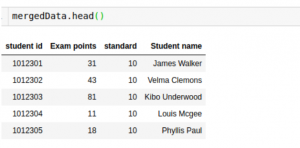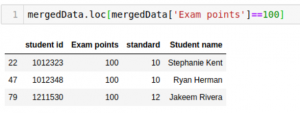Gyan Prakash Tripathi — February 5, 2020

## The Challenge of Merging Multiple Dataframes in Python

Here’s a scenario that trips up almost every fresher and aspiring data scientist:

You are working on a project where data is being collected from several sources. Before you can get to the exploring and model-building part, you would need to first join these multiple datasets (in the form of tables, dataframes, etc.). How can you do this without losing any information?

This might sound like a simple scenario but it can be intimidating for a lot of newcomers, especially those who are unfamiliar with Python programming.Drilling down further into this, I can broadly classify this into two scenarios:

1. First, the data with similar attributes may be distributed into multiple files. For example, suppose you are provided with multiple files each of which stores the information of sales that occurred in a particular week of the year. Thus, you will have 52 files for the whole year. Each file will have the same number and names of the columns.
2. Second, you may require combining information from multiple sources. For example, let’s say you want to get the contact information of people who have bought your products. Here you have two files – the first one with sales information and a second one with information about the customers.

I will show you how to work with both scenarios and join multiple dataframes in Python.

## Understanding the Problem at Hand

I’ll take a popular and easy-to-understand example for the purpose of this article.

Let’s consider the example of examinations in a particular school. There are various subjects being taught with different teachers assigned to each subject. They update their own files regarding the student marks and overall performance. We’re talking about multiple files here!

For this article, we will use two such files that I have created to demonstrate the working of functions in Python. The first file contains data about class 12th students and the other one has data for class 10th. We will also use a third file that stores the names of students along with their Student ID.

Note: While these datasets are created from scratch, I encourage you to apply what you’ll learn on a dataset of your choice.

## Step-by-Step Process for Merging Dataframes in Python

Here’s how we’ll approach this problem:

1. Load the Datasets in Python
2. Combine Two Similar Dataframes (Append)
3. Combine Information from Two Dataframes (Merge)

We will use three separate datasets in this article. First, we need to load these files into separate dataframes.

The first two dataframes contain the percentage of students along with their Student ID. In our first dataframe, we have the marks for class 10 students while the second dataframe contains marks for the students in 12th standard. The third dataframe contains the names of students along with their respective Student ID.

We can use the ‘head’ function to check the first few rows of each dataframe:## Step 2: Combining Two Similar Dataframes (Append)

Let’s combine the files of class 10th and 12th in order to find the average marks scored by the students. Here, we will use the ‘append’ function from the Pandas library:

Output: ((50,3),(50,3),(100,3))

As you can see from the output, the append function adds the two dataframes vertically.

The resultant dataframe is allMarks. The shapes of all three dataframes are compared above.

Next, let’s have a look at the content of ‘allMarks’ and calculate the mean:Output: 49.74

## Step 3: Combining Information from Two Dataframes (Merge)

Now, let’s say we want to find the name of the student who came first among both the batches. Here, we do not need to add the dataframes vertically. We will have to scale it horizontally in order to add one more column for the name of students.

To do this, we will find the maximum marks scored:

Output: 100

The maximum marks achieved by a student are 100. Now, we will use the ‘merge’ function to find the name of this student:Finally, the resultant dataframe has names of students mapped along with their marks.

The merge function requires a necessary attribute on which the two dataframes will be merged. We need to pass the name of this column is in the ‘on’ argument.

Another important argument of merge is ‘how’. This specifies the type of join you want to perform on the dataframes. Here are the different join types you can perform (SQL users will be very familiar with this):

• Inner join (performed by default if you don’t provide any argument)
• Outer join
• Right join
• Left join

We can also sort the dataframe using the ‘sort’ argument. These are the most commonly used arguments while merging two dataframes.

Now, we will see the rows where the dataframe contains 100 ‘Exam points’:Three students have got 100 marks, out of which two are in class 10th. Well done!

## End Notes

Pretty straightforward, right? No need to trip yourself up over this anymore! You can go ahead and apply this to any dataset of your choice. My recommendation is to pick up the food forecasting challenge that contains 3 different files.

If you are a newcomer to Python for data science, you can enroll in this free course.###### Gyan Prakash Tripathi

Builds and Tells Stories with Data. Gyan works out Data Science Projects at Analytics Vidhya. He loves to dig out interesting results and build up stories from data-heaps. He is very passionate about exploring rising technologies.